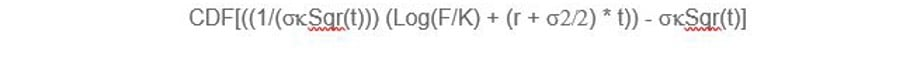# Understanding the CME OPEC Watch Tool and Expected Outcomes Calculator

• 13 Jun 2022
• By CME Group
• Topics: Energy

## CME OPEC Watch Tool assumptions and interpretations

• OPEC+ meeting outcome probabilities for the June 30, 2022 meeting are calculated from the LOQ2 monthly contract and the nearest active LO weekly option prices.
• An implied volatility curve for a generated June 30 expiration is interpolated from these available option expirations.
• The polynomial method is used to derive an implied probability distribution from the generated June 30 expiration. A centering reference future price is calibrated to the arithmetic average of the last five settlement prices of CL1 from June 9 (21 days to expiration) from the valuation date.
• The ATM (at-the-money) implied volatility from the generated June 30 expiration is priced, and a 1-standard deviation range is calculated from that implied volatility and time to expiration.
• The 1-standard deviation range is recalibrated at 14, 7, and 4 days to expiration (DTE).
• The 1-standard deviation range is applied to the centering reference future price.
• Once the CME OPEC Watch Tool starts tracking, an implied distribution will be derived in real time from the generated June 30 expiration:
• The probability area ≥ +1 SD price will be the ‘No Change or Small Output Decrease’ %
• The probability area ≤ -1 SD price will be the ‘Further Output Increase’ %
• The probability area > -1 SD and < +1 SD will be the ‘Maintain Output Increase’ %

## Methodology:

There are different methods for extracting probabilities from options prices. A number of these involve estimating a probability density function (PDF) by inverting an options pricing formula such as Black-Scholes and using option market prices to solve for the pricing density function. Most theoretical option pricing models rely on an assumption of lognormally distributed prices, or normally distributed log returns. These models assume this standard normal density function applies to value an option of any strike price for a given expiration. Observed futures price returns, however, may not conform to a standard normal distribution. Observed returns may be skewed or kurtotic, meaning the realized price distributions could have greater probabilities of extreme price moves and tend to cluster more returns around current futures price levels versus these models. The effect on option prices in the market is for volatility to vary by strike price. Instead of the same volatility for each option strike, ‘implied volatilities’ will differ by strike. These strike and time-varying implied volatilities imply a probability density function of futures returns that may have greater skew or kurtosis than a standard normal.

It is possible to estimate this non-standard normal probability density function by inverting theoretical value equations and solving for an implied probability distribution that fits current option market prices. Note, different methods can yield slightly different implied probabilities as more than one density function can satisfy current option prices, given the same constraints. The method used for the CME OPEC Watch Tool probabilities is as follows:

D N (d2)/D Moneyness

Where Moneyness is defined as Log(K/F)/Sqr(dte)

1. Implied volatilities are calculated and fitted by a fitting function (of the form x^n) from current option and futures prices on the LOQ2 contract and from the nearest active LO weekly option prices. An implied volatility curve for a generated June 30 expiration is interpolated from these available option expirations.
2. From the implied volatility function, the value N (d2) is calculated by strike (k), where N (d2) is defined as:3. The ATM (at-the-money) implied volatility from the generated June 30 expiration is priced, and a 1-standard deviation range is calculated from that implied volatility and time to expiration.
4. The 1-standard deviation range is recalibrated at 14, 7, and 4 days to expiration (DTE).
5. The 1-standard deviation range is applied to the centering reference future price.
6. The implied event probabilities are then set as:
• The probability area ≥ +1 SD price will be the ‘No Change or Small Output Decrease’ %
• The probability area ≤ -1 SD price will be the ‘Further Output Increase’ %
• The probability area > -1 SD and < +1 SD will be the ‘Maintain Output Increase’ %

See the Probabilities tab on the CME OPEC+ Watch tool for a table of the implied distribution calculation values.

## Disclaimer

All examples in this tool are hypothetical interpretations of situations and are used for explanation purposes only. The views in this tool reflect solely those of the author and not necessarily those of CME Group or its affiliated institutions. This tool and the information herein should not be considered investment advice or the results of actual market experience.  CME Group expressly disclaims all liability for the use or interpretation (whether by visitor or by others) of information contained herein. Decisions based on this information are the sole responsibility of the visitor.  The visitor agrees to hold the CME Group and its affiliates and licensors harmless against any claims for damages arising from any decisions that the visitor makes based on such information. Nothing contained in this tool constitutes investment advice, nor does it constitute the solicitation of the purchase or sale of any futures or options.

As the world’s leading derivatives marketplace, CME Group is where the world comes to manage risk. Comprised of four exchanges - CME, CBOT, NYMEX and COMEX - we offer the widest range of global benchmark products across all major asset classes, helping businesses everywhere mitigate the myriad of risks they face in today's uncertain global economy.

Follow us for global economic and financial news.